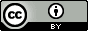## Wednesday, July 15, 2015

### Does this experiment measure ΔG° or ΔA°?

2016.01.02: update.  The experimental conditions are irrelevant.  What matters is that $K$ related to the change in standard chemical potential $\Delta \mu^\circ = \Delta G^\circ = \Delta A -RT^\circ$ and .  More info here

I recently came across this interesting paper from 1974 in which they measure the equilibrium constants for hydrogen bond formation between methanol and amines in the gas phase.  I find it interesting because it's a concrete example the practical considerations that underlying thermodynamics - in particular whether the experiment best approximates constant pressure or constant volume.

Adapted from Millen & Mines J. Chem. Soc., Faraday Trans. 2, 1974,70, 693-699. (c) Royal Society of Chemistry,  Reproduced with permission.

Here's a picture of the experimental set-up.  What you don't see is that "The entire apparatus was immersed in a glass-fronted thermostat controlled within $\pm$0.1 $^\circ$C."  In essence you have two containers A and B connected by a valve C, which, initially is closed.  You know the volume of container A ($V_{\rm{A}}$) and B ($V_{\rm{B}}$) from calibration experiments using nitrogen gas for some level of mercury in the manometers.

To start an experiment you fill container A with some gas (e.g. methanol) by briefly opening valve D and you do the same for some amine in container B but you make sure that the pressure in B is higher than that in A. (I'll call the molecules in container A, $A$ and similarly for B.) Then you let the system sit a while so that the temperature of the gas is the same as the thermostat.  Then you record the equilibrium pressures in container A ($p_{\rm{A,i}}$) and B ($p_{\rm{B,i}}$) by reading off the heights of the mercury columns (to within $\pm$0.05 mm Hg) using a cathetomer.  The increase in volume due to the mercury moving can be calculated using the radius of the manometer tube and added to the measure volumes to yield $V_{\rm{A,i}}$ and $V_{\rm{B,i}}$

Then you open valve C briefly and let some gas flow from B into A, wait for equilibration, and re-measure the pressure in A ($p$) and B ($p_{\rm{B, f}}$).  The pressure in volume A
$$p=p_A+p_B+p_{A_2}+p_{B_2}+p_{AB}$$
is a sum of the pressures of the individual species now present in container A, such as molecule $A$, dimers of $A$ ($A_2$), etc. This expression can be rewritten as
$$p=p_A+p_B+K_{A_2}p_A^2+K_{B_2}p_B^2+K_{AB}p_Ap_B$$
where $K_{AB}$ is the equilibrium constant we are after.

$K_{A_2}$ and $K_{B_2}$ can be measured by similar experiments on pure $A$ and and $B$, but we have two additional unknowns $p_A$ and  $p_B$ so we need two additional equations:
$$\pi_A=p_A+2K_{A_2}p_A^2 +K_{AB}p_Ap_B$$
$$\pi_B=p_B+2K_{B_2}p_B^2 +K_{AB}p_Ap_B$$
Here $\pi_A$ is the "formal" pressure of $A$ which is the "the pressure the compound would exert if present in the vapour phase solely as monomer obeying the ideal gas law", and similarly for $B$.

The initial formal pressure of $A$ can be computed from the initial pressure measurement ($p_{\rm{A,i}}$) and the second virial coefficient of $A$ ($B_A(T)$)
$$\pi_{A,\rm{i}} = \frac{p_{\rm{A,i}}}{1+B_A(T)/V_{\rm{A,i}}}$$
and from this we can compute the final formal pressure of $A$ using Boyle's law.
$$\pi_A = \frac{\pi_{A,\rm{i}}V_{\rm{A,i}}}{V_{\rm{A,f}}}$$
$V_{\rm{A,f}} \ne V_{\rm{A,i}}$ because the level of mercury in the manometer changes but $\Delta V_{\rm{A}}$ can easily be computed knowing the radius of the manometer tube.

To get the formal pressure of $B$ in container A we convert the initial and final pressures measured for container B to formal pressures and compute the number of moles of $B$ transferred to volume A
$$\Delta n_B = \frac{\pi_{\rm{B,i}}V_{\rm{B,i}}-\pi_{\rm{B,f}}V_{\rm{B,f}}}{RT}$$
and use this value to compute the formal pressure of $B$ in volume A, $\pi_B$
$$\pi_B = \frac{\Delta n_B RT}{V_{\rm{A,f}}}$$
What about the standard free energy change?
Colloquially speaking, if we use the raw pressure data $K_{AB}$ will have units of (mm Hg)$^{-1}$ but after we convert it to bar$^{-1}$ we can compute a standard free energy change from $K_{AB}$. The question is whether this free energy change corresponds to a Gibbs ($\Delta G^\circ$) or Helmholtz free energy ($\Delta A^\circ$) change or something in between.  The Gibbs free energy corresponds to constant pressure while the Helmholtz free energy corresponds to constant volume, and neither seem to strictly apply. The short answer is "I don't know" while the long answer is:

I think the standard free energy change most closely represents $\Delta A^\circ$
While volumes A and B are connected under equilibrium conditions, $K_{AB}$ represents equilibrium measurements done on both volumes A and B, so the thermodynamic system is comprised of both volumes.  The manometer tubes most likely have the same radius to maximize error cancellation, so the decrease in volume B would be quite similar to the increase in volume A, leading to a small net volume change for the system.

What do you think?